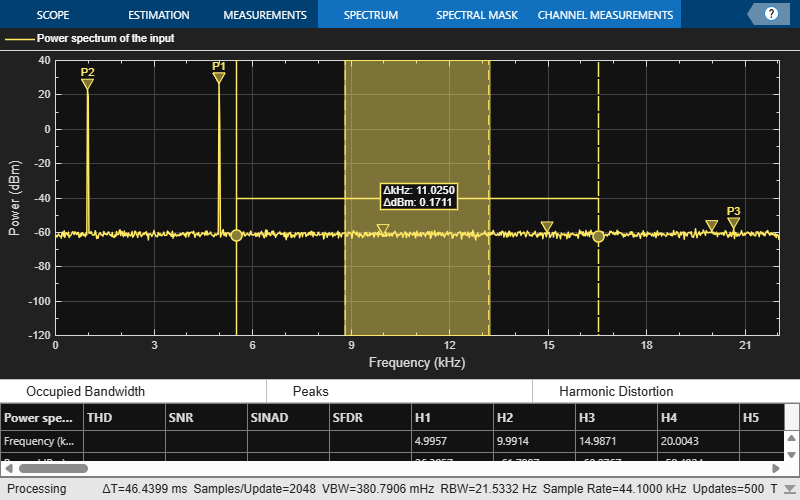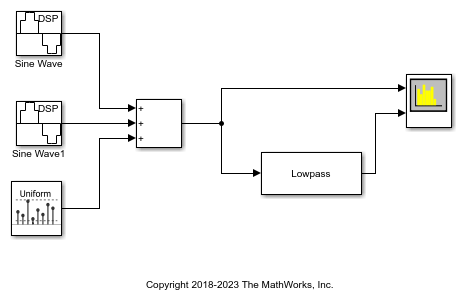getMeasurementsData

Get the current measurement data displayed on the spectrum analyzer

Description

example

data = getMeasurementsData(scope) returns a data table about the current spectrum analyzer measurements in use.

data = getMeasurementsData(scope,'all') returns a data table about all spectrum analyzer measurements for the current time step.

Examples

collapse all

Compute and display the power spectrum of a noisy sinusoidal input signal using the dsp.SpectrumAnalyzer System object™. Measure the peaks, cursor placements, adjacent channel power ratio, distortion, and CCDF values in the spectrum by enabling the following properties:

• PeakFinder

• CursorMeasurements

• ChannelMeasurements

• DistortionMeasurements

• CCDFMeasurements

Initialization

The input sine wave has two frequencies: 1000 Hz and 5000 Hz. Create two dsp.SineWave System objects to generate these two frequencies. Create a dsp.SpectrumAnalyzer System object to compute and display the power spectrum.

Fs = 44100;
Sineobject1 = dsp.SineWave('SamplesPerFrame',1024,'PhaseOffset',10,...
'SampleRate',Fs,'Frequency',1000);
Sineobject2 = dsp.SineWave('SamplesPerFrame',1024,...
'SampleRate',Fs,'Frequency',5000);
SA = dsp.SpectrumAnalyzer('SampleRate',Fs,'Method','Filter bank',...
'SpectrumType','Power','PlotAsTwoSidedSpectrum',false,...
'ChannelNames',{'Power spectrum of the input'},'YLimits',[-120 40],'ShowLegend',true);

Enable Measurements Data

To obtain the measurements, set the Enable property of the measurements to true.

SA.CursorMeasurements.Enable = true;
SA.ChannelMeasurements.Enable = true;
SA.PeakFinder.Enable = true;
SA.DistortionMeasurements.Enable = true;

Use getMeasurementsData

Stream in the noisy sine wave input signal and estimate the power spectrum of the signal using the spectrum analyzer. Measure the characteristics of the spectrum. Use the getMeasurementsData function to obtain these measurements programmatically. The isNewDataReady function indicates when there is new spectrum data. The measured data is stored in the variable data.

data = [];
for Iter = 1:1000
Sinewave1 = Sineobject1();
Sinewave2 = Sineobject2();
Input = Sinewave1 + Sinewave2;
NoisyInput = Input + 0.001*randn(1024,1);
SA(NoisyInput);
data = [data;getMeasurementsData(SA)];
end
endThe right side of the spectrum analyzer shows the enabled measurement panes. The values shown in these panes match with the values shown in the last time step of the data variable. You can access the individual fields of data to obtain the various measurements programmatically.

Compare Peak Values

Peak values are obtained by the PeakFinder property. Verify that the peak values obtained in the last time step of data match the values shown on the spectrum analyzer plot.

peakvalues = data.PeakFinder(end).Value
peakvalues = 3×1

26.9850
24.1735
-52.3506

frequencieskHz = data.PeakFinder(end).Frequency/1000
frequencieskHz = 3×1

4.9957
0.9905
7.8166

Compute and display the power spectrum of a noisy sinusoidal input signal using the Spectrum Analyzer block. Measure the peaks, cursor placements, adjacent channel power ratio, distortion, and CCDF values in the spectrum by enabling these block configuration properties:

• PeakFinder

• CursorMeasurements

• ChannelMeasurements

• DistortionMeasurements

• CCDFMeasurements

Open and Inspect the Model

Filter a streaming noisy sinusoidal input signal using a Lowpass Filter block. The input signal consists of two sinusoidal tones: 1 kHz and 15 kHz. The noise is white Gaussian noise with zero mean and a variance of 0.05. The sampling frequency is 44.1 kHz. Open the model and inspect the various block settings.

model = 'spectrumanalyzer_measurements.slx';
open_system(model)Access the configuration properties of the Spectrum Analyzer block using the get_param function.

sablock = 'spectrumanalyzer_measurements/Spectrum Analyzer';
cfg = get_param(sablock,'ScopeConfiguration');

Enable Measurements Data

To obtain the measurements, set the Enable property of the measurements to true.

cfg.CursorMeasurements.Enable = true;
cfg.ChannelMeasurements.Enable = true;
cfg.PeakFinder.Enable = true;
cfg.DistortionMeasurements.Enable = true;

Simulate the Model

Run the model. The Spectrum Analyzer block compares the original spectrum with the filtered spectrum.

sim(model)The right side of the spectrum analyzer shows the enabled measurement panes.

Using getMeasurementsData

Use the getMeasurementsData function to obtain these measurements programmatically.

data = getMeasurementsData(cfg)
data =

1x5 table

SimulationTime    PeakFinder    CursorMeasurements    ChannelMeasurements    DistortionMeasurements
______________    __________    __________________    ___________________    ______________________

{[0.9985]}      1x1 struct        1x1 struct            1x1 struct               1x1 struct

The values shown in measurement panes match the values shown in data. You can access the individual fields of data to obtain the various measurements programmatically.

Compare Peak Values

As an example, compare the peak values. Verify that the peak values obtained by data.PeakFinder match with the values seen in the Spectrum Analyzer window.

peakvalues = data.PeakFinder.Value
frequencieskHz = data.PeakFinder.Frequency/1000
peakvalues =

26.9113
26.4002
-5.0427

frequencieskHz =

15.0015
1.0049
13.1783

Save and Close the Model

save_system(model);
close_system(model);

Input Arguments

collapse all

Spectrum analyzer you want to query. Specify a dsp.SpectrumAnalyzer System object or a SpectrumAnalyzerConfiguration object for a spectrum analyzer block.

Output Arguments

collapse all

When you specify 'all', a measurements table containing the following fields is returned:

FieldDescription
SimulationTimeSimulation time
PeakFinderPeak finder data
CursorMeasurementsCursor measurements data
ChannelMeasurementsChannel measurements data
DistortionMeasurementsDistortion measurements data
CCDFMeasurementsCCDF measurements data

When you do not specify 'all', the data table contains only the spectrum analyzer measurements currently in use.

Blocks

Introduced in R2018b

DSP System Toolbox DocumentationGet trial now